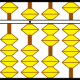# Regents Recap — January 2013: Miscellaneous Grievances

Here is another installment in my series reviewing the NY State Regents exams in mathematics.

The January 2013 math Regents exams contained many of the issues I’ve complained about before:  lack of appreciation for the subtleties of functions, asking for middle terms,  non-equivalent equivalent expressions, and the like.

I’ve chronicled some of the larger issues I saw this January here, but there were a few irritating problems that didn’t quite fit elsewhere.  Like number 9 from the Geometry exam.First of all, I don’t really understand why we bother writing multiple choice questions about constructions instead of just having students perform constructions.  Setting that issue aside, this question is totally pointless.

The triangle is equilateral.  Regardless of how it was constructed, the fact that AB = AC = BC will always justify its equilateralness.  Under no circumstance could the fact that  AB = AC = BC not justify a triangle is equilateral.  The construction aspect of this problem is entirely irrelevant.

Next, I really emphasize precise use of language in math class.  In my opinion, in order to think clearly about mathematical ideas, you need to communicate clearly and unambiguously about them.  The wording of number 32 from the Algebra 2 / Trig exam bothers me.What does the answer mean in the phrase “express the answer in simplest radical form”?  Presumably it means “the two solutions to the equation”, but “answer” is singular.  And if it means “the set of solutions”, well, you can’t put a set in simplest radical form.

Are we trying to trick the students into thinking there’s only one solution?  Or is this just a lazy use of the word “answer”, like the way students lazily use the word “solve” to mean dozens of different things?  I understand that this is nit-picking, but this is a particular pet peeve on mine.

Lastly, number 20 from the Geometry exam is simply absurd.  Just looking at it makes me uncomfortable.I’m sure we can find a better way to test knowledge of logical relationships than by promoting common mathematical errors!

Categories: Teaching#### Joshua Bowman (@Thalesdisciple) · March 11, 2013 at 11:13 am#### Thy Tran · March 11, 2013 at 2:46 pm

But I’m a bit confused as to the exception you take with “equilateralness” (#9). You say “the construction aspect of this problem is entirely irrelevant”. Perhaps I’m being too generous with the test writer here but I interpreted the question to mean: how do you justify *this* particular construction. That is, if one does the construction shown using pencil and compass, how does one know that one has constructed an equilateral triangle? The answer is: “by construction, AB=AC=BC”.#### MrHonner · March 11, 2013 at 8:07 pm

I’m sure that’s exactly what the question writer meant.

However, regardless of which specific sequence of constructions is used to produce an equilateral triangle, the sides produced will be congruent. This fact then justifies that an equilateral triangle has been constructed.

In fact, there really isn’t any way other than showing the sides are congruent to justify that a triangle is equilateral. That is, after all, the definition of equilateral.#### CCSSIMath · March 11, 2013 at 5:36 pm

Ambiguous wording is a perennial issue in math tests, and Common Core’s move towards more scenario questions is going to make it worse, but our biggest issue with #9 is that the skills, properties and theorems involved–constructing an equilateral triangle, properties of equilateral triangles, the triangle angle sum theorem, and the triangle inequality theorem–all belong in elementary school.

http://ccssimath.blogspot.com/2012/11/mathematical-tools-part-1.html#### Amy · March 11, 2013 at 11:20 pm

#20. Now that’s embarrassing.#### JBL · April 1, 2013 at 11:57 pm

Christ what an embarrassment. Numbers 20 and 32, while obviously terrible questions, at least are decipherable, but how could you possibly justify calling (2) a wrong answer for number 9? If I can show those angles are equal, that would certainly (together with a standard theorem) justify the claim that the triangle is equilateral! I would be ashamed to give questions like this to my students, let alone every 10th grader in NY.

Follow